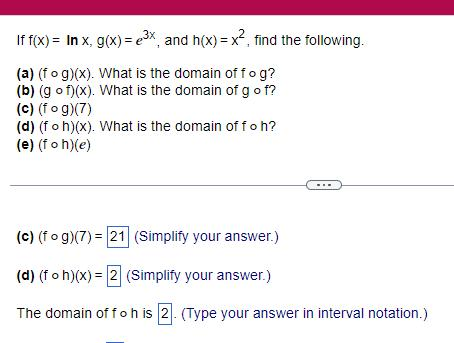Question:

# If f x In x g x e x and h x x find the following a fog x

Last updated: 11/21/2023If f x In x g x e x and h x x find the following a fog x What is the domain of fog b gof x What is the domain of g o f c fog 7 d foh x What is the domain of f o h e foh e c fog 7 21 Simplify your answer d f oh x 2 Simplify your answer The domain of fo h is 2 Type your answer in interval notation